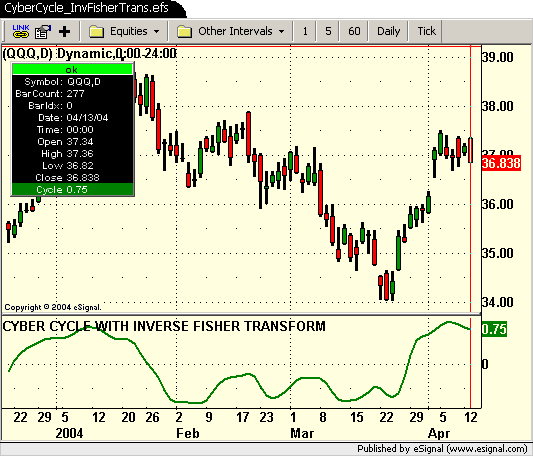# 2004 May: CyberCycle_InvFisherTrans.efs

ICE Data Services -

 CyberCycle_InvFisherTrans.efs

File Name: CyberCycle_InvFisherTrans.efs

Description:
Based on The Inverse Fisher Transform by John Ehlers. This article appeared in the May 2004 issue of Stock & Commodities.

Formula Parameters:
alpha - 0.07

Notes:
The related article is copyrighted material. If you are not a subscriber of Stocks & Commodities, please visit www.traders.com.
The May 2004 EFS formulas were provided by TS Support.
The current version was modified by eSignal for real time intraday analysis 4/20/2004.`// Magazine: Technical Analysis of Stocks & Commodities, May 2004// Article: The Inverse Fisher Transform by John F. Ehlers// Study: CYBER CYCLE WITH INVERSE FISHER TRANSFORM// Provided By: TS Support, LLC for eSignal// Version 1.1 4/20/2004 Modified by eSignal for real time analysisvar Smooth = 0;var Smooth_1 = 0;var Smooth_2 = 0;var Cycle = 0;var Cycle_1 = 0;var Cycle_2 = 0;function preMain(){ setStudyTitle("CYBER CYCLE WITH INVERSE FISHER TRANSFORM"); setCursorLabelName("Cycle",0); setDefaultBarFgColor(Color.green,0); setDefaultBarThickness(2); //setComputeOnClose();}function main(alpha){ if(alpha == null) alpha = .07; var ICycle = 0; if (getBarState() == BARSTATE_NEWBAR) { Smooth_2 = Smooth_1; Smooth_1 = Smooth; Cycle_2 = Cycle_1; Cycle_1 = Cycle; } Smooth = ((high() + low()) / 2 + high(-1) + low(-1) + high(-2) + low(-2) + (high(-3) + low(-3)) / 2 ) / 6; Cycle = (1 - .5 * alpha) * (1 - .5 * alpha) * (Smooth - 2 * Smooth_1 + Smooth_2) + 2 * (1 - alpha) * Cycle_1 - (1 - alpha) * (1 - alpha) * Cycle_2; if(getCurrentBarIndex() - getOldestBarIndex() < 7) Cycle = ((high() + low()) / 2 - high(-1) - low(-1) + high(-2) + low(-2)) / 4; ICycle = (Math.exp(2 * Cycle) - 1) / (Math.exp(2 * Cycle) + 1); return ICycle;}`